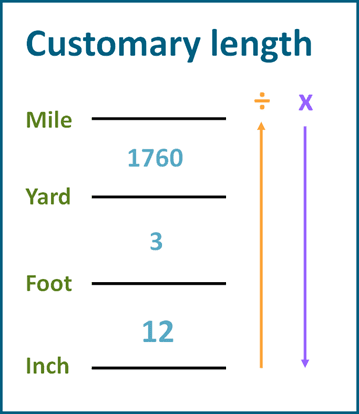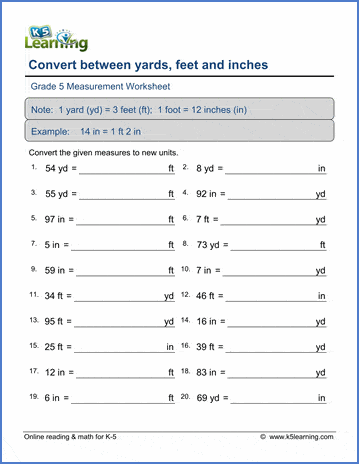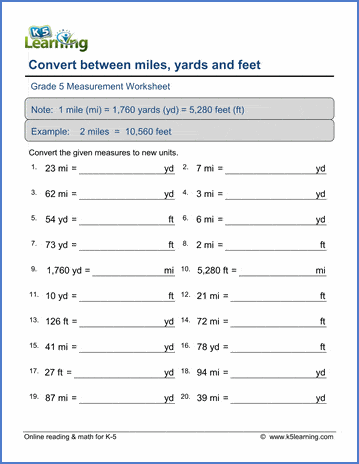# Converting Lengths between Miles, Yards, Feet and Inches

For measuring length, the US customary system uses inches, feet, yards and miles.

## How big are the US customary units?

A quarter is about 1 inch.

A man's shoe is about 1 foot.

A guitar is about 1 yard.

A runway at a large airport is about 1 mile.

## Converting US customary units

In grade 5, students practice converting between different measurements units for length. Let us show you how.

## Converting larger units to smaller units

To convert the larger units to smaller units, we multiply the number of large units by the amount of the smaller unit. The units convert as follows:

1 mile = 1,760 yards

I yard = 36 inches

1 yard = 3 feet

1 foot = 12 inches

For example:

Convert 4 feet to inches.

4 x 12 = 48 inches.

## Converting smaller units to larger units

To convert smaller units, we divide the number of smaller units by the larger unit’s conversion factor, as follows:

1 inch = 1÷12 foot

1 foot = 1÷3 yard

1 yard = 1÷1,760 mile

For example:

Convert 72 inches to feet.

72÷12 = 6 feet

Here’s a handy chart that summarizes how to convert customary units of length:## Practice converting between inches, feet and yards

In our grade 5 measurement section, we have a series of worksheets to help students practice converting between inches, feet and yards.## Grade 5 worksheets to practice converting between miles, feet and yards

Similar to the above worksheets, students now practice converting between miles, feet and yards.